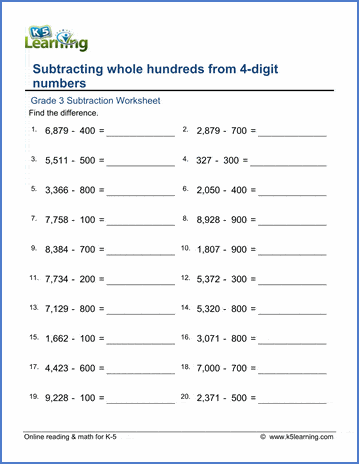# Subtracting hundreds from 4-digit numbers

## Worksheets: Subtracting whole hundreds (100, 200, ... ,900) from 4-digit numbers

Below are six versions of our grade 3 math worksheet on subtracting whole hundreds under 1,000 from random 4-digit numbers.  This exercise combines simple mental subtraction with consideration of place value concepts. These worksheets are pdf files.## More subtraction worksheets

Explore all of our subtraction worksheets, from subtracting by counting objects to subtracting large numbers in columns.

## What is K5?

K5 Learning offers reading and math worksheets, workbooks and an online reading and math program for kids in kindergarten to grade 5.  We help your children build good study habits and excel in school.